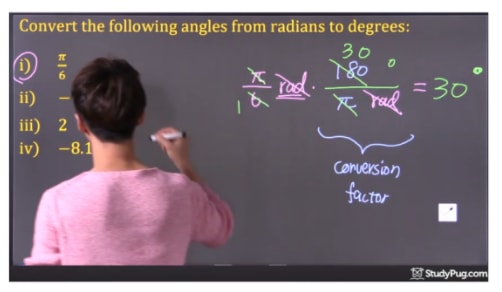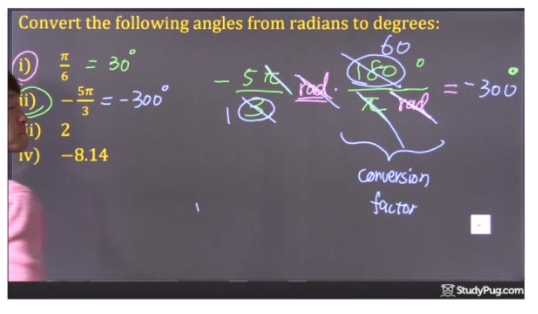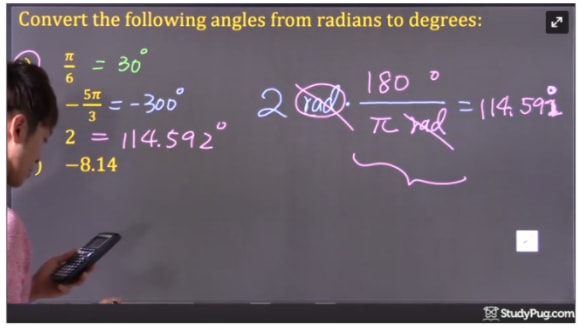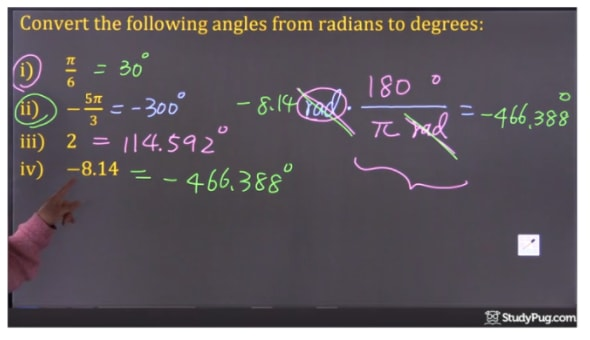# Converting between degrees and radians

## What is a radian?

A radian is a type of unit used for measuring angles. The other unit that you're probably more familiar with when it comes to measuring angles is degrees. While there's more than two units that help depict the measure of an angle, radian and degrees are the two that you'll have to deal with most.

You're probably wondering why we have to use radians when we already have degrees. That is because degrees are not actually numbers. In order to compute math, we need to work with numbers. A good example that's similar to this concept is using decimals when we have percentages. Although a percentage can be shown with a number followed by a % sign, when we do math, we convert that to a decimal (or fraction).

In this lesson, we'll learn how to convert radians to degrees, and also the other way around: turning degrees into radians.

## Converting radians to degrees

To start off, let's first convert radians into units that we're more familiar with: degrees.

Question

Convert the following angles from radians to degrees

1. i) $\frac{\pi }{6}$

Solution

Same as converting other units, when converting radians to degrees, we need to know the conversion factor. In a sense, this is the radians to degrees formula that can help you change from one unit to another. In the case of converting radian to degree, the conversion factor is $\frac{{180^\circ }}{{\pi \;rad}}$ .

Now, we can multiply $\frac{\pi }{6}$ with the conversion factor $\frac{{180^\circ }}{{\pi \;rad}}$ . The $\pi$ and rad cancel out each other, and then only $\frac{{30^\circ }}{1}$ is left. So, the final answer is $30^\circ$Convert pi/6 to 30 degree
1. ii) $- \frac{{5\pi }}{3}$

Similar to the first part of the question, we multiply $- \frac{{5\pi }}{3}$ with the conversion factor $\frac{{180^\circ }}{{\pi \;rad}}$. Again, the $\pi$ and rad cancel out each other.  Both The 3 and the $180^\circ$ are divided by 3. We have $- \frac{5}{1} \bullet \frac{{60^\circ }}{1}$ left, and we will then multiply them and get the final answer $- 300^\circ$Convert -5pi/3 to -300 degree

iii) 2

We multiply 2 with the conversion factor $\frac{{180^\circ }}{{\pi \;rad}}$. The rad cancels out each other and leaves $2 \bullet \frac{{180^\circ }}{\pi }$. Using the calculator, we are able to get the final answer $114.592^\circ$Convert 2 radian to degree

iii) -8.14

We multiply -8.14 with the conversion factor $\frac{{180^\circ }}{{\pi \;rad}}$. The rad cancels out each other and leaves us with $- 8.14 \bullet \frac{{180^\circ }}{\pi }$. Using the calculator, we are able to get the final answer $- 466.388^\circ$Convert -8.14 radian to degree

## Converting degrees to radians

Conversely, we can convert degrees to radians. Let's take a look at these sets of questions.

Question

Convert the following angles from degrees to radians

1. i) $85^\circ$

Solution

The conversion factor for converting degrees to radian is $\frac{{\pi \;radian}}{{180^\circ }}$

We can multiply $85^\circ$ with $\frac{{\pi \;radian}}{{180^\circ }}$. The degree signs cancel out each out. We have $85 \bullet \frac{{\pi \;radian}}{{180}}$ left. Multiplying them with a calculator, we get the final answer 1.48 radian.

Similar questions can be done in the same way as this method we just explored:

Multiply the degrees with conversion factor $\frac{{\pi \;radian}}{{180^\circ }}$ . Use calculator for the multiplication when it is necessary. We will then get the final answer.

As an online resource, if you wanted to quickly convert between radians and decimals, use this online converter to help you out or double check your work.

### Converting between degrees and radians

#### Lessons

180° = $\pi$ radians
• 1.
Review "unit conversion"
a)
24 roses = ___________ dozens of roses

b)
3 dozens of roses = ___________ roses

c)
65 kg = __________ lbs

d)
100 lbs = __________ kg

• 2.
Convert the following angles from degrees to radians
a)
85°

b)
-213°

c)
30°

d)
60°

e)
45°

f)

g)
90°

h)
180°

i)
270°

j)
360°

• 3.
Convert the following angles from radians to degrees
a)
$\pi \over 6$

b)
$-{ {5\pi} \over 3}$

c)
2

d)
-8.14